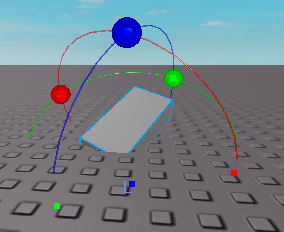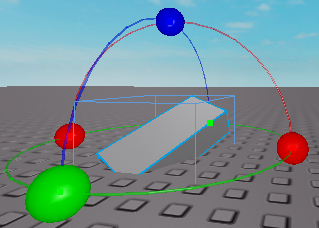# How do you rotate a CFrame on the global axis?

What I’m trying to do is rotate a CFrame on the global axis. The current issue is that it only rotates locally. How would I be able to rotate my CFrame globally?
Example of Local Rotation:Example of Global Rotation:I need it rotated only with CFrame. I don’t need to rotate it using .Orientation.

use CFrame:ToWorldSpace

How would I use that with rotations?

you can put a cframe in it
like

``part.CFrame = part.CFrame:ToWorldSpace(CFrame.Angles(69,420,3.14))``

How would I do it with two CFrame.Angles?

what do you mean by that?

Like if you were doing CFrame.Angles(23,123,123)*CFrame.Angles(321,123,52)

that multiplication still returns in a cframe.angles then
i think you can just straight putting it in or make it a var

What exactly do you mean by that?

You can

``````part.CFrame = part.CFrame:ToWorldSpace(CFrame.Angles(69,420,3.14)*CFrame.Angles(1,33,7))
``````

or

``````local cframethingies = CFrame.Angles(69,420,3.14)*CFrame.Angles(1,33,7)
part.CFrame = part.CFrame:ToWorldSpace(cframethingies)
``````

right?

Both of those didn’t rotate the part properly.

if not rotated in the expected rotation use math.rad

I used math.rad for it though.

t h e
sorry
beyond what i know

If you meant in the workspace, you can toggle local and global by doing CTRL + L.

Otherwise you just multiply the cframe as an offset to what you’re trying to rotate by

Something like
`part.CFrame = CFrame.Angles(0, math.pi, 0) * part.CFrame`
`part.CFrame = part.CFrame * CFrame.Angles(0, math.pi, 0)`

nvm i just tested this, diddd nott work out like i pictured it in my head, has some purpose though.

ok here is what i had in mind:
`script.Parent.CFrame = CFrame.Angles(0, rot, 0) * (cf - cf.Position) + cf.Position`
where cf is script.Parent.CFrame, this works for my case at least.

1 Like

You’d want to take the global cframe and convert it to object space, so you’d still be rotating the object locally, but with a cframe that matches the global cframe you want to use (see CFrame:ToObjectSpace() on the wiki). Then you’d just apply the cframe like you would locally.

``````local part = script.Parent
local cframethingies = CFrame.Angles(0,0,math.rad(5))
part.CFrame = part.CFrame:ToObjectSpace(cframethingies)
``````

So like that?

``````local part = script.Parent
local globalCF = CFrame.Angles(0,0,math.rad(5))
local objectSpaceCF = globalCF:ToObjectSpace(part.CFrame)
part.CFrame = part.CFrame * objectSpaceCF
``````

is how I think you’d implement it

I tried that out, but it didn’t work with all of the axis. How do I make it so it works with each axis?

That way didn’t work. It was orientated really weirdly.